[中文] Search Internet Search HelloDBA
 Home Tech Documents Products & Download Products >> Sharing About
Oracle Technology Site. email: fuyuncat@gmail.com  MSN: fuyuncat@hotmail.com# Full Table Scan cost formula cracking(1)

[中文]

Author:  fuyuncat

Source:  www.HelloDBA.com

Date:  2009-08-01 15:03:37

Share to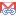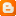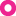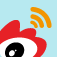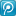# 1.Preface

CBO is a relatively more accurate optimizer, it will choose the access path considering more objective factors, to draw a more reasonable execution plan. However, Oracle does not give us complete formula for calculating the cost, so we do not know the impact of these factors in the costs calculation. When we try to adjust some parameters, there is no formula that can be relied upon. However, Oracle provides a method of CBO and the output of the Trace (10053). This is for us the equivalent of a "black box", we are able to enter some data to the black box, and it will return the output.
Many of us have probably done some IQ test questions, of which a very typical questions that are given a set of figures, but among the missing 1,2, and let you out of this by observing the law of numbers to supplement the lack of figures. For example, a group of figures 1,2,? , 5,8,? , 21, through observation, this group of figures can be such a law of Xn = Xn-1 + Xn-2, in accordance with this rule, we can draw the two missing figures were 3,13.
Then we can be black-box input and output to try to deduce how the black box in the end of this operation?

# 2.Background

CBO is Cost Based Optimizer, therefore, calculating the cost of query plan is the core of CBO. From Oracle Concept, as well as many other documents, we know a number of factors of the cost of query plan. For example, data block number under the HWM, the number of records in the table, distinct value number of the column, index height, the host CPU processing power and so on. In Metalink, we can find a given to formula COST:

Cost = (

#SRds * sreadtim +

#MRds * mreadtim +

#CPUCycles / cpuspeed

) / sreadtim

The factors are:

• # SRDS: single data block reads;
• # MRDS: multiple data blocks reads;
• SREADTIM: single data block read time;
• MREADTIM: multiple data blocks read time;
• # CPUCYCLES: CPU cycles
• CPUSPEED: CPU processing speed

However, this formula is not enough to help us calculate the cost of a query plan. Because, you can see, except CPUSPEED is a data can be get freely (from System Statistics to obtain or by calculate CPU speed multiplied by CPU number), the other factors need more information to be calculated (in the workload model, SREADTIM, MREADTIM can be obtained, but in noworkload model, the two data still need to calculate).

Fortunately, there are experts gave detail explanation on the above formula. Jonathan Lewis described how to calculate # SRDS, # MRDS in his book, as well as how to calculate SREADTIM and MREADTIM in noworkload mode:
# SRDS = BLEVEL + INDLEAFBLKS * INDSEL + TABSEL * CLUF (index scan)
# MRDS = TABBLKS / MBRC (full table scan, fast full index scan)
SREADTIM = IOSEEKTIM + BLKSIZ / IOTFRSPEED
MREADTIM = IOSEEKTIM + MBRC * BLKSIZ / IOTFRSPEED

Among them,

• BLEVEL: index level;
• INDLEAFBLKS: the number of index leaf block;
• INDSEL: index selectivity;
• TABSEL: Table selectivity;
• TABBLKS: number of data blocks under the table in the HWM;
• MBRC: Multi_Block_Read_Count;
• BLKSIZ: data block size;
• IOSEEKTIM: IO seek time, default is 10ms;
• IOTFRSPEED: IO transfer speed, default is 4096 bytes / ms.

We can see that, except INDSEL and TABSEL, all data can be directly obtained (about INDSEL and TABSEL, Oracle also has given the formula, but it is not complete, I will try to complete it in another article). So far, except # CPUCYCLE , all of the factors in the former formula can be calculated. Unfortunately, in the Oracle documents and Jonathan Lewis's book, we did not find how # CPUCYCLE be calculated. The job I will do is to try to derive the formula of calculating the # CPUCYCLE. If we can successfully derive the formula of # CPUCYCLE, we can further derive other formula by the similar method.

Note: By the principle of  from simple to complex, I will derive the formula of # CPUCYCLE in Full Table Scan under NOWORKDLOAD System Statistics with bind variables. If we get it, we can derive other more complex formula. My database is Oracle10gR2.

Fortunately, we can get CBO's  trace file by 10053 event. The10053 trace file logged the whole process of optimizer to generate optimal (minimum cost) execution plan, including the relevant basic information, query rewriting, calculation on the related factors, and so on. The following trace file is something we are interested in:

1. PARAMETERS USED BY THE OPTIMIZER

``***************************************``
``PARAMETERS USED BY THE OPTIMIZER``
``********************************``
``  *************************************``
``  PARAMETERS WITH ALTERED VALUES``
``  ******************************``
``  parallel_execution_enabled          = false``
``  pga_aggregate_target                = 0 KB``
``  optimizer_mode                      = choose``
``  optimizer_index_cost_adj            = 60``
``  *************************************``
``  PARAMETERS WITH DEFAULT VALUES``
``  ******************************``
``  optimizer_mode_hinted               = false``
``  optimizer_features_hinted           = 0.0.0``
``… …``

2. SYSTEM STATISTICS INFORMATION

``*****************************``
``SYSTEM STATISTICS INFORMATION``
``*****************************``
``  Using NOWORKLOAD Stats``
``  CPUSPEED: 1000 millions instruction/sec``
``  IOTFRSPEED: 4096 bytes per millisecond (default is 4096)``
``  IOSEEKTIM: 10 milliseconds (default is 10)``

3. BASE (Table, Index, Columns) STATISTICAL INFORMATION

``***************************************``
``BASE STATISTICAL INFORMATION``
``***********************``
``Table Stats::``
``  Table: T_TEST1  Alias: T_TEST1``
``    #Rows: 47582  #Blks:  673  AvgRowLen:  93.00``
``Index Stats::``
``  Index: T_TEST1_IDX1  Col#: 1``
``    LVLS: 1  #LB: 109  #DK: 22  LB/K: 4.00  DB/K: 58.00  CLUF: 1277.00``
``  Index: T_TEST1_IDX2  Col#: 2 1``
``… …``

4. ACCESS PATH calculation

``***************************************``
``SINGLE TABLE ACCESS PATH``
``  Table: T_TEST1  Alias: T_TEST1     ``
``    Card: Original: 47582  Rounded: 47582  Computed: 47582.00  Non Adjusted: 47582.00``
``  Access Path: TableScan``
``    Cost:  150.95  Resp: 150.95  Degree: 0``
``      Cost_io: 149.00  Cost_cpu: 23349709``
``      Resp_io: 149.00  Resp_cpu: 23349709``
``… …``

The information is enough to help us to deduce the formula to get these results!

Look at the first part. It records the optimizer-related parameters. These data can affect the cost of query rewriting and calculation. Here, we are interested in some of data who will impact on the cost computing. We need understand how they effect in the formula in following deriving process.

In the second part, there is the system statistics information, including IOSEEKTIM, CPUSPEED, IOTFRSPEED and IOSEEKTIM we mentioned. There is 2 kind of System Statistic modes, one is workload mode, which requires to call dbms_stat to collect system statistics data automatically. Besides those 4 data, it will also collect the MREADTIM, SREADTIM, MBRC and other data. The other is noworkload mode. When the data are not available, or incomplete/incorrect, the noworkload mode will be used. Trace file will prompt us which mode be used currently. We can query the data from sys.aux_stats\$. These data can also be manually set by dbms_stat. In the deriving process, , I will modify these data by this package.

In the third part is the statistical information related to the objects. The meaning of the abbreviation can be found in the trace file header. Here is the explanation:

• Table

# Rows: the number of records in table;

# Blks: Table data blocks number below the HWM;

AvgRowLen: Average row length of table records

• Index

Col #: Column position in the index;

LVLS: index height, means, BLevel;

# LB: Index leaf block number;

LB/K: the average index leaf block number per key;

DB/K: the average data block number per key;

CLUF: Clustering Factor

• Column

(# n): column position;

A (VARCHAR2): column name and data type;

AvgLen: the average length of the column;

NDV: the distinct value number of the columns;

Nulls: Null value number of the columns;

Density: the column density;

The fourth part is the process to calculate the cost of access path. What we need to pay attention to is COST_CPU, that is, # CPUCYCLES. Our method is to modify the data in previous three parts, observed changes of the data in this part, to find the rule and derive formula.

According to mathematical induction, we can proof a formula through the two steps: when x = 1, prove f (x) is correct; assume that f (n) is correct, prove f (n +1) is also correct. However, as the process is a black box process, unable to complete the second step. I will take a method no so accurate: Input a number of random data and get the output, if there is any result calculated by the derived formula is not same as the output, we said the formula is wrong, otherwise, it will be accepted.

In judicature, there are two kinds of conviction methods, one is called innocence conclusion, and the other is called guilt conclusion. Innocence conclusion is to assume the suspect is innocent, the prosecution need to find sufficient evidence to prove the suspect guilty, otherwise, he/she will be innocent; In the guilt conclusion, the prosecution has not sufficient evidence, but assume the suspects guilty, and the suspect must find evidence to prove him/her is innocence, otherwise he/she will be guilty. Despite guilty conclusion is unfair, but the method we adopt here is similar to guilt conclusion.

# 3.Process

Here we go! For easily finding the rule purpose, I will set related data to be tens, to avoid the rounding:

``SQL> create table t_peeking11 (a varchar2(50), b varchar2(50), c varchar2(50), d varchar2(80), e varchar2(50));``
`` ``
``表已创建。``
`` ``
``SQL> begin``
``  2   dbms_stats.set_system_stats(pname => 'CPUSPEEDNW',pvalue => 1000);``
``  3   dbms_stats.set_system_stats(pname => 'IOSEEKTIM',pvalue => 10);``
``  4   dbms_stats.set_system_stats(pname => 'IOTFRSPEED',pvalue => 4096);``
``  5   dbms_stats.set_table_stats(ownname => 'DEMO', tabname => 'T_PEEKING11',``
``  6                              numrows => 1000000, numblks => 1000, avgrlen => 100);``
``  7   dbms_stats.set_column_stats(ownname => 'DEMO', tabname => 'T_PEEKING11', colname => 'A',``
``  8                               distcnt => 1600, density => null, nullcnt => 0, avgclen=> 50);``
``  9   dbms_stats.set_column_stats(ownname => 'DEMO', tabname => 'T_PEEKING11', colname => 'B',``
`` 10                               distcnt => 1600, density => null, nullcnt => 0, avgclen=> 50);``
`` 11   dbms_stats.set_column_stats(ownname => 'DEMO', tabname => 'T_PEEKING11', colname => 'C',``
`` 12                               distcnt => 1600, density => null, nullcnt => 0, avgclen=> 50);``
`` 13   dbms_stats.set_column_stats(ownname => 'DEMO', tabname => 'T_PEEKING11', colname => 'D',``
`` 14                               distcnt => 1600, density => null, nullcnt => 0, avgclen=> 50);``
`` 15   dbms_stats.set_column_stats(ownname => 'DEMO', tabname => 'T_PEEKING11', colname => 'E',``
`` 16                               distcnt => 1600, density => null, nullcnt => 0, avgclen=> 50);``
`` 17  end;``
`` 18  /``
`` ``
``PL/SQL 过程已成功完成。``

Then generate a trace file as baseline:

``SQL> alter session set events '10053 trace name context forever,level 1';``
`` ``
``会话已更改。``
`` ``
``SQL> explain plan for select /*+full(a)*/* from T_PEEKING11 a where a > :v1;``
`` ``
``已解释。``
`` ``
``SQL> alter session set events '10053 trace name context off';``
`` ``
``会话已更改。``

Note: In the following process, for comparison purpose, I also created other test tables as baseline to derive certain factors, and some special data may be not same as the data here (such as the number of columns, records, etc.). But it does not affect the derived formula.
Note: The following sequence of derivation is not the actual derivation sequence. When I work on some factor, such as _optimizer_block_size, found it will not directly impact on the formula, until other factor is derived, and I will back to process on them again. The following order I set is for easy reading.